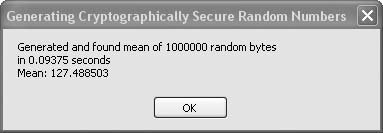# Recipe 16.8. Generating Cryptographically Secure Random Numbers

#### Problem

You want to generate reliably unpredictable pseudorandom bytes.

#### Solution

Sample code folder: Chapter 16\RandomNumbers

Use the RNGCryptoServiceProvider class provided in the System.Security.Cryptography namespace to generate random numbers that are guaranteed to be unpredictable and highly resistant to any pattern analysis.

#### Discussion

Some random number generators, such as those found in Visual Basic 6.0 and earlier versions of BASIC, were not really that good. They generally were fine for most statistical analysis purposes, but their cycle lengths were comparatively short, and certain types of high-powered random number tests showed them to have subtle patterns in the bits comprising their sequences of bytes. The RNGCryptoServiceProvider class provides a random number generator that's been carefully studied by professional cryptographers and passes all the standard tests for randomness with flying colors. There's no realistic way to analyze or predict the next byte in a sequence generated by this class.

The following code demonstrates the RNGCryptoServiceProvider class by using an instance of it to generate a million random bytes. The mean of these bytes is calculated, as is the time it takes to generate the bytes:

` Dim result As New System.Text.StringBuilder Const ProcessSize As Integer = 1000000 ' ----- Generate the random content. Dim randomEngine As New RNGCryptoServiceProvider( ) Dim randomBytes(ProcessSize) As Byte Dim timeStart As Date = Now randomEngine.GetBytes(randomBytes) ' ----- Calculate the mean of all values. Dim mean As Double For counter As Integer = 1 To ProcessSize     mean += randomBytes(counter) Next counter mean /= ProcessSize ' ----- How long did this take? Dim timeElapsed As Double = _    Now.Subtract(timeStart).TotalSeconds ' ----- Display the results. result.AppendLine(String.Format( _    "Generated and found mean of {0} random bytes", _    ProcessSize)) result.AppendLine(String.Format("in {0} seconds", _    timeElapsed)) result.Append("Mean: " & mean) MsgBox(result.ToString( )) `

The results for a sample run appear in Figure 16-7. You can call the GetBytes() method to fill any size byte array you pass to it with that many random bytes. The previous code generates the million bytes using only one call to the GetBytes() method. The loop processes the individual byes to calculate the mean.

##### Figure 16-7. Cryptographically secure random bytes generated by the RNGCryptoServiceProvider classBecause the random bytes have equal probabilities for all values from 0 to 255, the average value should theoretically be very near 127.5. With a million random bytes generated by this sample code, the mean falls very close to this theoretical value almost every time.Visual Basic 2005 Cookbook: Solutions for VB 2005 Programmers (Cookbooks (OReilly))
ISBN: 0596101775
EAN: 2147483647
Year: 2006
Pages: 400